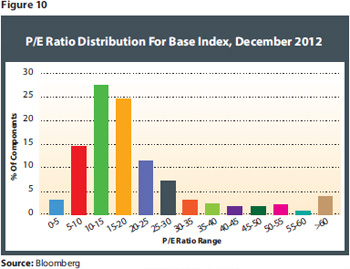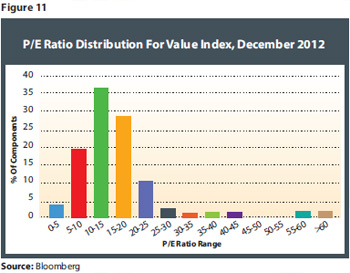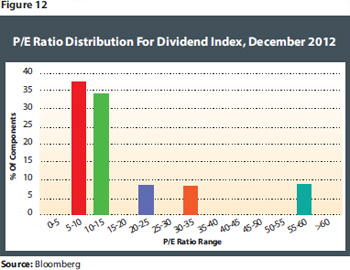# Journal Of Indexes: Dividends Vs. Value

March 13, 2014

Fundamentals Of Value Investing

But what about the other way around? Can the relatively simple and easy-to-follow dividend strategy be considered a valid proxy for the more complex and less-easy-to-follow value approach? To determine this while keeping a consistent approach, we need to examine the resulting portfolios in terms of the value definition employed by the value selection process. The value selection scheme considers multiple fundamental factors, which we will examine one by one, using the same distribution charts as shown before.

The first selection criterion is the price-to-earnings ratio, which is used to ensure overpriced stocks are not included in the index. We would therefore naturally expect price-to-earnings ratios to be lower in the value portfolio than in the base portfolio. And indeed, the average price-to-earnings ratio drops from 24.06 to 18.89 in the value portfolio. The analysis of the distributions shows that the selection mechanism successfully eliminates the higher price-to-earnings ratios from the index portfolio, and shifts the distribution significantly further to the left, with over 80 percent of P/E ratios falling below 20. See Figures 10 and 11.Turning to the dividend-based approach, the concept actually further reduces the average price-to-earnings ratio to 12.97, representing a decrease of almost 50 percent. Also, the resulting distribution of the results is similar to that of the value index. The dividend screening produces similar results in terms of price-to-earnings ratios to the value-based screening.

From Figure 12, we may assume that selection by dividend yield can be considered an equally effective measure as using price-to-earnings ratios directly to determine components with low valuations in this criterion. One may of course argue that this is hardly surprising given that the price component in both key figures is the same, and dividends may well be considered a proxy for earnings strength. While this is of course true, the results still show that inconsistencies between dividend payments and actual earnings do not seem to dilute or hurt this selection process.